< BACK TO BLOG

# Immutable vs Mutable Objects in Python

Published 17 April, 2019

Everything in Python is an object, even integers, booleans, floats, strings etc…

Objects can be divided in two major sections: immutable and mutable, but what are the differences between these two fancy terms?

In this post I’m going to show you the differences between immutable and mutable by diving into Python objects and knowing them well enough.

## Everything is an object

To prove that everything is an object in Python, go ahead and run the following snippet:

``````print( type(1) ) # Output: <class 'int'>
print ( type(1.2) ) # Output: <class 'float'>
print( type("Hello") ) # Output: <class 'str'>
``````

This snippet shows us that 1 is a class of type int, whereas 1.2 is a class of type float and "Hello" is a class of type str.

Try the other types like boolean, and you’ll get the same result.

`type()` is a Python built-in function in which it accepts a single parameter as an object and it returns the type of this object.

## Object Identifier

When we instantiate an object, Python allocates a memory address for it and gives it a unique id at the runtime, and once it’s set it can never change.

By using the id() function we can retrieve the object id at the runtime:

``````a = "Hello"
print( id(a) ) # Output: a random number
``````

Just remember that the object id is the location of the object in the memory.

## Immutable

Let’s demystify immutable objects by an example:

``````h1 = "Hello"
h2 = "Hello"

print( id(h1) ) # Output: random number like: 4545012936
print( id(h2) ) # as same id(1)
``````

As you see here, both h1 and h2 have the same id, but why? It’s because they are using the same object location.

Once "Hello World" is defined, it’ll get its own unique id and whenever we use the same string "Hello World" over and over, Python will only allocate one address in memory for it: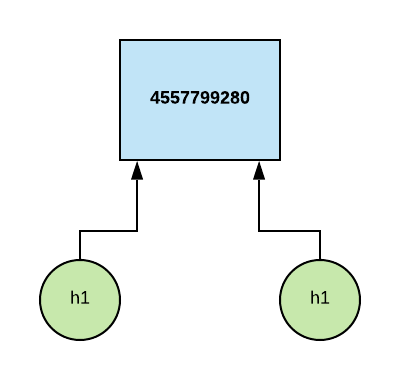Both objects (`h1` and `h2`) referencing the same memory allocated id.

So, regardless of how many times we define the "Hello World" string, we’ll get exactly the same object id.

Let’s dig a bit deeper and examine the location of the "Hello World" string:

``````h1 = "Hello World"
h2 = "Hello World"

print( id(h1) ) # Output: 4368067376 (random number)
print( id(h2) ) # Output: same as id(h1)
print( id("Hello World") ) # Output: same as h1 and h2
``````

Yes, "Hello World" has exactly the same id as h1 and h2.

In short, h1 and h2 are immutable, which means their values cannot be change at runtime, rather using the same object id over and over.

Let’s take another example:

``````h1 += ", I love Python"
print(h1) # Output: Hello World, I love Python
``````

t seems like we are appending a stirng into h1, in fact we are not changing the object that which h1 referencing to, instead, we are creating a new object with a value of "Hello World, I love Python":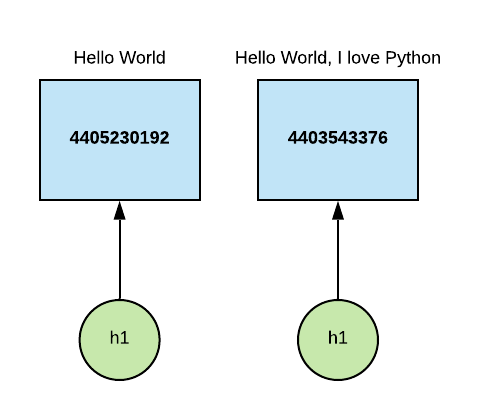Notice that the `h1` got a new object id.

Behind the scenes, Python creates and manages all the objects for us, so "Hello World" is an object by itself as well as "Hello World, I love Python".

Python will automatically get rid of "Hello World" by using its garbage collector, because it’s no longer needed.

Fair enough, now it’s the time to talk about the assignment operator.

The assignment operator (=) binds an object to a name, it does not copy any values, for example name = "Ahmad" means it binds the name to the object "Ahmad", so "Ahmad" has its own unique id, this unique id won’t be changed during runtime:

``````name  = "Ahmad"
name1 = "Ahmad"
name2 = "Ahmad"
name3 = "Ahmad"

# All these statements print the same value
print( id(name) )
print( id(name1) )
print( id(name2) )
print( id(name3) )
``````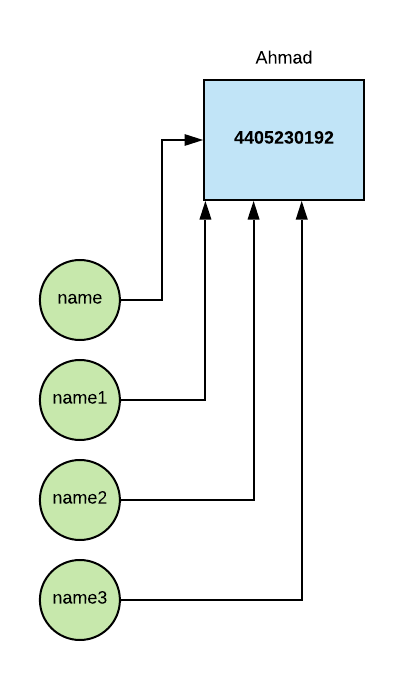Regardless of how many times “Ahmad” is used, it has one address in memory.

All the data types in Python are immutable except list, dict and set.

Hold a sec, where are the variables here? why didn’t I mention the term variable?

Python doesn’t really have variables in the metaphorical sense of a box holding a value. It only has named references to objects, and these references behave more like labels which allow us to retrieve objects.
Cite: Python Apprentice by Robert Smallshire and Austin Bingham (https://leanpub.com/python-apprentice) (Chapter 4 – Built-in types and the object model).

## Mutable

So far, we discussed the immutable objects, now time to discuss the mutable objects.

In mutable, the value can be changed during runtime without affecting the object id, so the object id (which is the memory address) remains intact:

Let’s examine mutable by an an example where we append a new item into a list:

``````s1 = [1, 2, 3, 4]
s2 = s1
s2.append(5)
print(s1) # Output: [1, 2, 3, 4, 5]
``````

You might ask why s1 returns the whole list? shouldn’t it return list from 1 to 4 instead of 1 to 5?

No, that because any changes we make to either s1 or s2 will affects the object reference: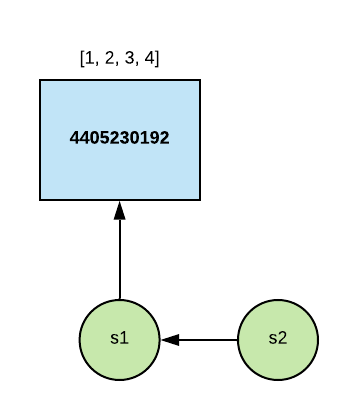`s2` has the same id as `s1`.

So, by changing s2 we are changing the reference itself, and by doing so, both s1 ands2 will be affected, since they are referencing the same object: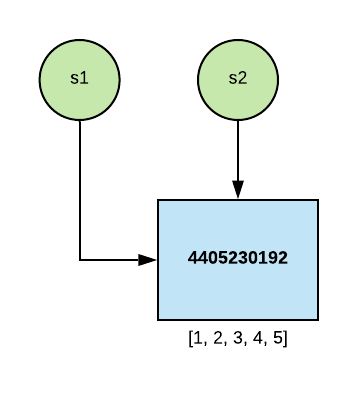s1 and s2 are using the exact same object.

In contrast with immutable, mutable objects will always have different ids at runtime, let’s take a look at another example:

``````s1 = [1, 2, 3, 4]
s2 = [1, 2, 3, 4]

# s1 and s1 have different reference ids
print( id(s1) )
print( id(s2) )
``````

That’s all about mutable and immutable.

## Equality of value vs equality of identity

Let’s dig a bit deeper into == and is and see the differences between these two operators.

is tests whether two references refer to the same object, in other words it tests the identity equality:

``````a = "Hello"
b = "Hello"
print( a is b ) # Output: True
``````

That’s True, because "Hello" is an immutable hence its value will never change.

In other side, the == tests the value and it does not care about the object id at all:

``````s1 = [1, 2, 3, 4]
s2 = [1, 2, 3, 4]

print( s1 is s2 ) # Output: False
print( s1 == s2 ) # Output: True
``````

Since list is an mutable object, it gets a new id each time we create one, so of course s1 is s2 gives us False because they are using different ids while s1 == s2 gives us True because their values are identical.

## Conclusion

We discussed both immutable and mutable objects by using a bunch of examples, just a recap of what we’ve learnt:

• All objects in Python are immutable except list, dict and set which are mutable.
• id() function is used to reterive the object identifier in the memory.
• type() function is used to get the data type of a given object.
• is() tests the quality of two object identifier.
• == tests the value of two objects.

Edit on Github